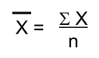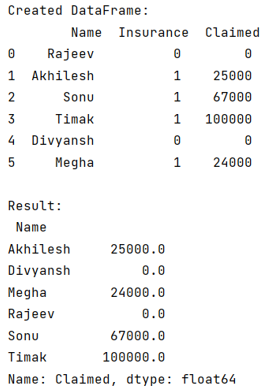# Python - How to calculate mean values grouped on another column in Pandas?

Given a Pandas DataFrame, we have to calculate mean values grouped on another column in Pandas.
Submitted by Pranit Sharma, on July 28, 2022

Pandas is a special tool that allows us to perform complex manipulations of data effectively and efficiently. Inside pandas, we mostly deal with a dataset in the form of DataFrame. DataFrames are 2-dimensional data structures in pandas. DataFrames consist of rows, columns, and data.

The pandas.DataFrame.groupby() method is a simple but very useful concept in pandas. By using groupby, we can create a grouping of certain values and perform some operations on those values.

The pandas.DataFrame.groupby() method split the object, apply some operations, and then combines them to create a group hence large amount of data and computations can be performed on these groups.

Syntax:

```DataFrame.groupby(
by=None,
axis=0,
level=None,
as_index=True,
sort=True,
group_keys=True,
squeeze=NoDefault.no_default,
observed=False,
dropna=True
)
```

Parameter(s):

It takes several parameters, but here we will use 'dropna = False', setting this value as False will not drop the NaN values from the column while grouping the elements.

To calculate mean values grouped on another column in pandas, we will use groupby, and then we will apply mean() method.

The average of a particular set of values is the called mean of that set. Mathematically, it can be represented as:Pandas allow us a direct method called mean() which calculates the average of the set passed into it.

Let us understand with the help of an example,

## Python code to calculate mean values grouped on another column in Pandas

```# Import pandas package
import pandas as pd

# import numpy package
import numpy as np

# Creating a dictionary
d = {
'Name': ['Rajeev', 'Akhilesh', 'Sonu', 'Timak', 'Divyansh', 'Megha'],
'Insurance': [0, 1, 1, 1, 0, 1],
'Claimed':[0,25000,67000,100000,0,24000]
}

# Creating a Dataframe
df = pd.DataFrame(d)

# Display the dataframe
print('Created DataFrame:\n',df,"\n")

# Calculating mean on groupby
result = df.groupby('Name')['Claimed'].mean()

# Display result
print("Result:\n",result)
```

Output: